Next: Laying out a mesh Up: FT AS AN INVERTIBLE Previous: FT AS AN INVERTIBLE

## The Nyquist frequency

The highest frequency in equation (6),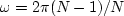,is almost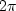.This frequency is twice as high as the Nyquist frequency.The Nyquist frequency is normally thought of as the highest possible'' frequency, because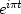, for integer t, plots as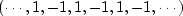.The double Nyquist frequency function,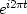, for integer t, plots as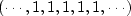.So this frequency above the highest frequency is really zero frequency! We need to recall that.Thus, all the frequencies near the upper end of the range (6) are really small negative frequencies. Negative frequencies on the interval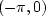were moved to interval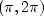by the matrix form of Fourier summation.

1Next: Laying out a mesh Up: FT AS AN INVERTIBLE Previous: FT AS AN INVERTIBLE
Stanford Exploration Project
10/21/1998